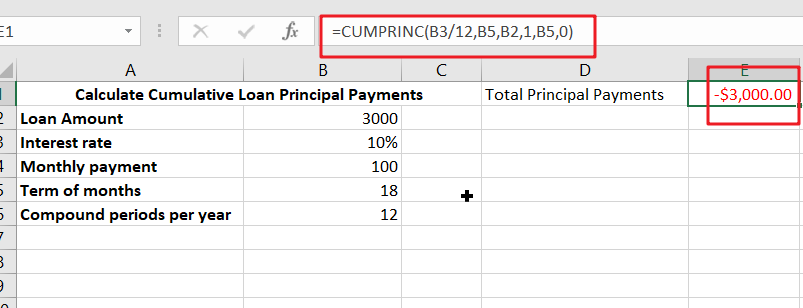# Calculate Cumulative Loan Principal Payments in Excel

How do you Calculate Cumulative Loan Principal Payments in Excel? There are a few different ways to calculate this information, but one way has been proven to be accurate more often than others. One option is to use the CUMPRINC formula in the cell. The result you get will be the total Principal Payments by the borrower over the loan’s lifetime.## Calculate Cumulative Loan Principal Payments Excel

You’ve likely wondered how to calculate the cumulative principal payment for your loan. This function returns the amount of principal paid over a period of time, such as the first quarter of each year. You should enter the period in months and use the CUMPRINC function to calculate this amount.

To calculate the cumulative principal payment for a loan, use the CUMPRINC function in Excel. First, you must enter the interest rate for each period. After you’ve entered the interest rate, you should calculate the number of periods for the total loan. Next, you must specify the total value of payments. You should also specify the start and end period for payments.

### Generic Formula

`=CUMPRINC(rate,nper,pv,start,end,type)`

### Summary:

You can also use the CUMPRINC function to determine the cumulative principal paid on a loan or investment in Excel. The CUMPRINC function takes four arguments: rate, nper, and PV, or start_period and end_period. The formula is:

`=CUMPRINC(B3/B6,B5,B2,1,B5,0)`

### Syntax:

• Rate: Interest rate for a specific period
• Nper: Total number of payments
• Pv: present value.
• Start_period: First period of interest
• End_period: last period of interest.

With these inputs, you can evaluate the original loan value!

### Explanation:

To calculate cumulative loan principal payments in Excel, you can use the CUMPRINC function. This function returns the total principal and interest you have paid so far. If you enter a negative value, you will receive an error. Make sure the start and end periods are equal before calculating the formula.

Once you have calculated the cumulative loan principal payments, you can adjust the length of your amortization schedule. For example, if you are making the last payments of a loan with a term of 10 years, you’ll need a formula for each year. The CUMPRINC function is available for use in Excel. It is a simple function that can be used in Excel to calculate loan principal payments and interest.

### Conclusion:

The CUMPRINC function calculates the cumulative amount of principal paid over a loan or investment period. The CUMPRINC function works best with a fixed interest rate and fixed payment schedule. To use the CUMPRINC function, enter the information for a payment period, which must be a number between one and the specified NPER.